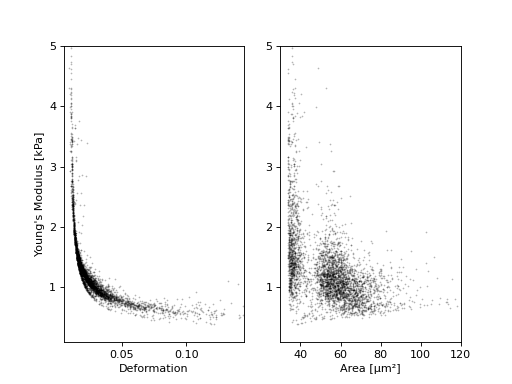# Young’s modulus computation¶

The computation of the Young’s modulus uses a look-up table (LUT) that was derived from finite elements methods [Mokbel2017] and the analytical solution [Mietke2015]. The LUT was computed using a linear elastic sphere model in an axis-symmetric channel (2D). The model computations take into account the equivalent channel radius for a square channel cross-section with the factor 1.094 (see also supplement S3 in [Mietke2015]). The original data used to generate the LUT are available on figshare [FigshareWittwer2020].

The computation takes into account corrections for the viscosity (medium, channel width, flow rate, and temperature) [Mietke2015] and corrections for pixelation of the area and the deformation which are computed from a (pixelated) image [Herold2017].

Since the Young’s modulus is model-dependent, it is not made available right away as an ancillary feature (in contrast to e.g. event volume or average event brightness).

```In : import dclab

In : ds = dclab.new_dataset("data/example.rtdc")

# "False", because we have not set any additional information.
In : "emodulus" in ds
Out: False
```

Additional information is required. There are three scenarios:

1. The viscosity/Young’s modulus is computed individually from the chip temperature for each event. Required information:
• The temp feature which holds the chip temperature of each event
• The configuration key [calculation]: ‘emodulus medium’
• The configuration key [calculation]: ‘emodulus model’
1. Set a global viscosity. Use this if you have measured the viscosity of your medium (and know all there is to know about shear thinning [Herold2017]). Required information:
• The configuration key [calculation]: ‘emodulus model’
• The configuration key [calculation]: ‘emodulus viscosity’
1. Compute the Young’s modulus using the viscosities of known media.
• The configuration key [calculation]: ‘emodulus medium’
• The configuration key [calculation]: ‘emodulus model’
• The configuration key [calculation]: ‘emodulus temperature’

Note that if ‘emodulus temperature’ is given, then this temperature is used, even if the temp feature exists (scenario A).

The key ‘emodulus model’ currently (2019) only supports the value ‘elastic sphere’. The key ‘emodulus medium’ must be one of the supported media defined in `dclab.features.emodulus_viscosity.KNOWN_MEDIA` and can be taken from [setup]: ‘medium’. The key ‘emodulus temperature’ is the mean chip temperature and could possibly be available in [setup]: ‘temperature’.

```import matplotlib.pylab as plt

import dclab

ds = dclab.new_dataset("data/example.rtdc")

# Add additional information. We cannot go for (A), because this example
# does not have the temperature feature (`"temp" not in ds`). We go for
# (C), because the beads were measured in a known medium.
ds.config["calculation"]["emodulus medium"] = ds.config["setup"]["medium"]
ds.config["calculation"]["emodulus model"] = "elastic sphere"
ds.config["calculation"]["emodulus temperature"] = 23.0  # a guess

# Plot a few features
ax1 = plt.subplot(121)
ax1.plot(ds["deform"], ds["emodulus"], ".", color="k", markersize=1, alpha=.3)
ax1.set_ylim(0.1, 5)
ax1.set_xlim(0.005, 0.145)
ax1.set_xlabel(dclab.dfn.feature_name2label["deform"])
ax1.set_ylabel(dclab.dfn.feature_name2label["emodulus"])

ax2 = plt.subplot(122)
ax2.plot(ds["area_um"], ds["emodulus"], ".", color="k", markersize=1, alpha=.3)
ax2.set_ylim(0.1, 5)
ax2.set_xlim(30, 120)
ax2.set_xlabel(dclab.dfn.feature_name2label["area_um"])

plt.show()
```